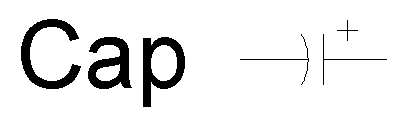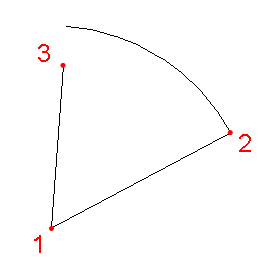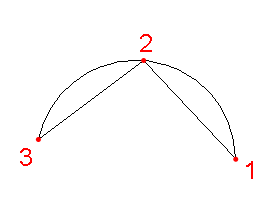Arc

Draw an arc using one of two methods.

Mode Settings:

Setting Data Type Comments
Method Radius, Angles | Three Points Choose method
Draw Clockwise Boolean Choose whether the angle extent of arcs is cw or ccw

Radius, Angles Method: Construct an arc from a center, radius, angle start and angle extent.

Prompt Data Type Comments
Center Point Center of the arc
Radius Point Specify radius and start angle simultaneously
Angle Extent Point or Angle Angle extent of the arcThree Points Method: Construct an arc from three points.

Prompt Data Type Comments
Point 1 Point
Point 2 Point
Point 3 PointInvalid State: Radius <= 0 | AngleExtent = 0